# Statistical Functions

The Statistical Functions blocks calculate statistical functions on a list of input values. See also: How to Add and Remove Input/Output Properties.

The following are accepted and excluded arguments for Statistical Functions blocks:

• Values of the input n property can be numbers, arrays, or references that contain numbers.
• Numbers, logical values, and numbers represented as strings are included in calculations.
• Input values are ignored if they are error values, null, or text that cannot be parsed as a number.
• Input values of zero are included in calculations.
Block Icon Description
Average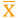Returns the mean of its arguments
Maximum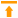Returns the greatest value in a list of arguments
Median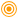Returns the median of its arguments
Minimum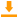Returns the smallest value in a list of arguments
Mode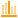Returns the most common value in a list of arguments
Standard Deviation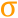Calculates standard deviation based on a list of arguments
Variance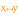Calculates the statistical variance of a list of arguments

2018/10/03 00:17